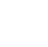Back to Chapter
Getting StartedBasic Syntax & DeclarationControl StatementsNo items found.
Function & EventsNo items found.
Array and its TypesNo items found.
OOP : Object Oriented ProgrammingNo items found.
Javascript StandardsNo items found.
HTML DOMNo items found.No items found.No items found.No items found.

# Introduction

Math is an Object that contains many mathematical properties and methods, you can use these to perform mathematical operations.

You Don't Need to Create Math Object First, because as it has no constructor so you can directly use it.

## Syntax for Accessing Mathematical Properties

``` Math.property ```

Properties

Example

``` console.log(Math.PI); // Output // 3.141592653589793 ```

## Syntax for Accessing Mathematical Methods

``` Math.methodName(number) ```

Methods

Example

``` console.log(Math.pow(4,2)); // Output // 16 ```

Here,

It will return \$2^4\$ i.e. 16.

## Math.random()

We have very important Math Function Math.random() that is used to return any random number between 0(included) to 1(excluded)

For Example : If you want to generate a random number Math.random() can be used.

### Syntax

``` Math.random(); ```

Example

``` console.log(Math.random()); //Return Number Between 0 to 1 console.log(Math.random() * 10); //Return Number Between 0 to 10 console.log(Math.random() * 100); //Return Number Between 0 to 100 console.log(Math.random() * 1000); //Return Number Between 0 to 1000 // Output // 0.7414042897047488 // 1.7638821680366013 // 65.38297196571448 // 614.161979173051 ```

Here,

These Random Outputs were generated in my program, you will be having different random number.